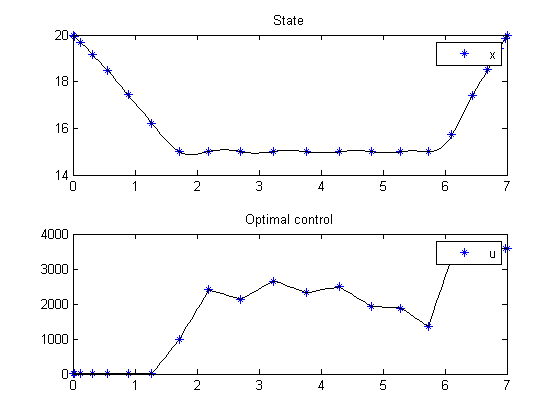110  Room temperature control

110.1  Problem Description

Temperature control from 0.00 to 7.00 hours at night. Finds best heating policy at night that brings the temperature back to 20 [oC] in the morning irrespective of night temperatures.

Programmers: Gerard Van Willigenburg (Wageningen University)

110.2  Problem setup

Define tomSym variable t (time) and t_f (final time) if the final time is free

toms t; t_f=7; % Fixed final time

for n=[20 40]
% Define & set time axis
p = tomPhase('p', t, 0, t_f, n);
setPhase(p);

% Define the state and control variables
tomStates x
tomControls u

% Initial state
xi=20;

% Initial guess
if n==20
x0 = {icollocate({x == xi(1)})
collocate({u == 0})};
else
x0 = {icollocate({x == xopt})
collocate({u == uopt})};
end

% Boundary conditions
cbnd = {initial({x == xi}); final({x == xi})};

% Equality constraints: state-space differential equations
tau=2; pH=0.002; % Parameters

% External input d1
d1=15-10*sin(pi*t/t_f);

%Differential equation
ceq = collocate({dot(x) == 1/tau*(d1-x) + pH*u});

% Inequality constraints
cbox = {0 <= collocate(u) <= 3600; icollocate(x) >= 15};

% Cost function to be minimized
objective = integrate(u+1e-6*dot(u)^2);

% Solve the problem after specifying its name
options = struct;
options.name = 'Temperature control at night';
solution = ezsolve(objective, {cbox, cbnd, ceq}, x0, options);

% Plot intermediate solution
figure; subplot(2,1,1);
ezplot(x); legend('x');
title('State');

subplot(2,1,2);
ezplot(u); legend('u');
title('Optimal control'); drawnow;

% Obtain intermediate solution to initialize the next
xopt = subs(x,solution);
uopt = subs(u,solution);
end

% Obtain final solution t,x,...,u,..
% that overwrite the associated tomSym variables
t = subs(collocate(t),solution);
x = subs(collocate(x),solution);
u = subs(collocate(u),solution);

%Plot results
figure; plot(t,x,t,u/100); axis([0 t_f -1 50]);
xlabel('Time [h]'); ylabel('Heat input, Inside & Outside temperature');
title('Optimal heating, outside temperature');
legend('Inside temp. [oC]','Outside temp. [oC]');
Problem type appears to be: qp
Starting numeric solver
===== * * * =================================================================== * * *
TOMLAB - Tomlab Optimization Inc. Development license  999001. Valid to 2011-02-05
=====================================================================================
Problem:  1: Temperature control at night       f_k   12892.776351482709000000
sum(|constr|)      0.000000000002029896
f(x_k) + sum(|constr|)  12892.776351482711000000
f(x_0)      0.000000000000000000

Solver: CPLEX.  EXIT=0.  INFORM=1.
CPLEX Barrier QP solver
Optimal solution found

FuncEv   11 GradEv   11 ConstrEv   11 Iter   11
CPU time: 0.062500 sec. Elapsed time: 0.031000 sec.
Problem type appears to be: qp
Starting numeric solver
===== * * * =================================================================== * * *
TOMLAB - Tomlab Optimization Inc. Development license  999001. Valid to 2011-02-05
=====================================================================================
Problem:  1: Temperature control at night       f_k   12882.457401725025000000
sum(|constr|)      0.000000006475484391
f(x_k) + sum(|constr|)  12882.457401731501000000
f(x_0)      0.000000000000000000

Solver: CPLEX.  EXIT=0.  INFORM=1.
CPLEX Barrier QP solver
Optimal solution found

FuncEv   13 GradEv   13 ConstrEv   13 Iter   13
CPU time: 0.062500 sec. Elapsed time: 0.047000 sec.